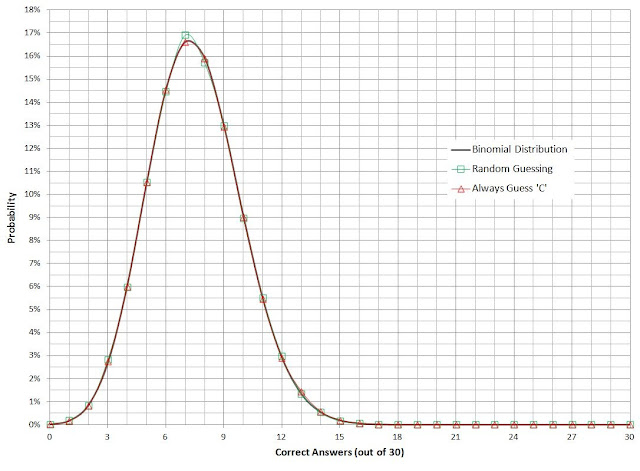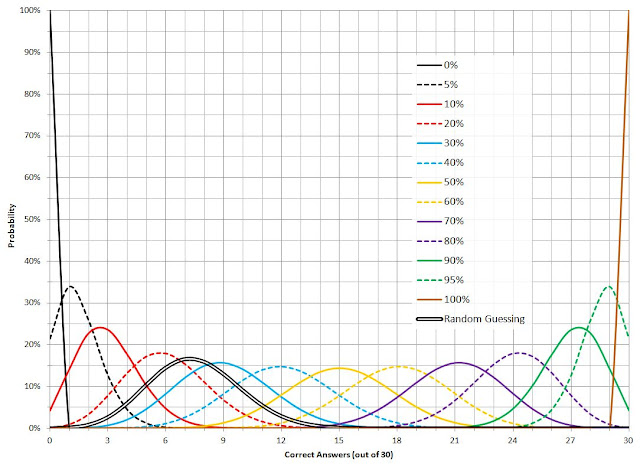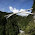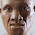Friday, 2 August 2013

Guessing on Multiple Choice Tests

You've probably heard someone give advice along the lines of "when in doubt, guess 'C'" when taking multiple choice tests. The alleged reasoning behind it is that there's a statistical advantage in guessing 'C' consistently rather than randomizing your answers.

I decided to investigate this claim. Let's first check the case when the professor distributes the correct answers evenly and randomly, not favouring any one letter. Take for example a test with 30 questions, where each answer is independent of the others and there are four choices per question (A, B,C, or D). Assuming that you really have no clue which is the correct answer, any guess has a 25% probability of being correct. This is true regardless of whether you choose randomly (let's ignore for the moment that humans are notoriously bad at generating random values on their own) or if you decide to choose the same letter consistently. Here's why:

The instructor randomly chooses which letter is correct, without bias, so the probability of any letter happening to be the correct answer on a particular question is 25%. If you also guess randomly without favouring any letter (i.e. each letter is guessed, on average, 25% of the time), you should then expect that 25% of the correct answers are 'A', 25% of your guesses are 'A', thus 6.25% of your guesses (25% of 25%) are correct. The same is true of 'B', 'C', and 'D', so that overall you expect 4*6.25% = 25% of your guesses to be correct. Now if you consistently choose 'C' for the same random test, the probability that 'A' is correct is 25% but the probability that you guess 'A' is 0%. This is also true of 'B' and 'D'. When you choose 'C' 100% of the time, overall you should expect that 3*0.25*0 + 1*0.25*1 = 25% of your guesses are correct.

Since the guess is either right or wrong, this is a problem for the binomial distribution. The mean is n*p and the variance is sqrt[n*p*(1-p)], where n is the number of trials and p is the probability of the desired outcome. In our example multiple-choice test, whether you guess randomly or choose 'C' every time, you would expect, on average, to get 30*0.25 = 7.5 correct answers. To reinforce the point that both approaches to guessing are equal here, I simulated 50,000 of these tests in Excel and generated the following plot.Probability distribution for correct guesses on tests with random answers to four-choice questions.
The average was 7.49 correct answers with a standard deviation of 2.37 when all guesses were random. When 'C' was guessed consistently, the average was 7.50 with a standard deviation of 2.38. The binomial distribution with n = 30 and p = 0.25 predicts an average of 7.50 and a standard deviation of 2.37. It's pretty clear that both guessing schemes give the same results when the correct answers are random and evenly distributed among the possible choices.

Now that it's clear that there's no advantage if answers are randomly and evenly distributed, let's investigate the case where the instructor favours one letter over the others. It turns out that no matter how biased the instructor's distribution of correct answers might be, if you randomly guess each letter 25% of the time, your probability of choosing the right answer is still 25%. Let's assign unknown probabilities for each letter being assigned as correct by the instructor: pA, pB, pC, and pD. The sum of these unknown probabilities must be unity. So when the probability of guessing A, B, C, or D is 25% each, the probability of being correct is then 0.25pA+0.25pB+0.25pC+0.25pD = 0.25(pA+pB+pC+pD) = 0.25*1 = 25%. That all changes if you consistently choose 'C' though. In this case, your probability of getting the right answer is 0pA+0pB+1*pC+0pD = pC. I varied pC between the most unfavourable case (where 'C' is never the correct answer) to the most favourable (where 'C' is always the correct answer) to generate the following plot:Probability distribution for correct answers when 'C' guessed consistently for different values of pC.
Obviously, there's some benefit to choosing 'C' if you know that the instructor favours 'C' over the other letters. But you also get screwed if the instructor doesn't like to use 'C' or simply chooses to favour another letter because he wants to penalize the people who always guess 'C'. While it looks pretty nice that more of the curves I plotted are shifted to the right rather than left of the curve for random guessing, if the choice of which letter gets favoured is random, the chance that 'C' is never the right answer is higher than the chance that it is always correct. To illustrate, I ran a simulation which I think is slightly more realistic than the examples above.

I assumed that pB + pC is 54% on average, which is just a guess on my part but I feel it is reasonable because answers aren't randomly assigned a letter. Numerical answers are often ordered from smallest to largest and statements like "All of the above" are reserved only for 'D' because they'd be confusing if they weren't. I used the normal distribution to generate the random variations in individual tests, so that pB + pC can vary from 0 to 1, but is usually close to the average. I similarly used normally distributed random numbers to split up pB and pC, so that on average pC is half of (pB + pC), but can vary from 0 to 100%. Same idea with pA and pD. Random guesses by the test taker are still equally distributed on average between the four choices. Plotted below are the results of 250,000 simulated tests.Probability distribution for correct answers based on "realistic" multiple choice tests.
With random guessing we expected to be right 25% of the time on average and to see a binomial distribution after many tests. When 'C' is guessed consistently, things get more complicated, but a simple approximation can be found using the normal distribution. As you can see in the plot, the approximation works fairly well. It is clear that random guessing gives you more consistent results than guessing 'C' all the time. Always guessing 'C' increases your chances of getting 1 in 3 guesses right, but it also increases your chances of doing worse than 1 in 6, simply because your results are influenced by how the distribution of answers was biased by the instructor for the particular test. Overall, consistently guessing 'C' resulted in 2% more correct answers. But of the 250,000 simulated tests, random guessing beat consistently guessing 'C' on a total of 125,568 tests (i.e 50.23% of the time).

To summarize, if you happen to know that the creators of the test favour 'C' for correct answers, guessing 'C' consistently gives you an edge. In the long run, sticking with 'C' probably gives you a very slim advantage over random guessing, though guessing randomly gives you better consistency in the results of your guesses.

1.Or you could look to see what letter has been used for other answers that you know are correct and extropolate which letter is being preferred, then use that as your constant guess... which means skip the questions you do not know until you answer those you do know. Then go back and guess, if necessary.

2.What you've described is essentially an example of the gambler's fallacy. In a small sample of random questions, it is likely that one answer will be selected more often than the others. However, all the correct answers are independent of each other (assuming that the test questions don't refer you back to the answers of previous questions). Assuming no bias by the instructor, if you're part way through a test and find that 1/3 of your answers are 'C', there's still no guarantee that 'C' is actually more likely to be correct. It might be a clue, but it could also just be how the random selections worked out. If you see one letter showing up more often, you'd have to perform a statistical analysis and decide if this observation is statistically significant. Constant guessing is only advantageous over random guessing when your choice really does have a higher probability of being correct. Therefore, you must be confident that there really is some bias in the test.

3.Answering C will fail True or False questions. Make sure you answer A or B on a True-Or-False.
Answering "all of the above", on questions that have "all of the above" as an answer, will likely be beneficial as well.

4.There are a lot of online apps and the courses that are helping the candidates to study better and that too without leaving the home. Even I love to study without any distractions so my room is just perfect place for that. This is why I have picked one of the best Mobile Bar Review Courses that is helping me immensely.